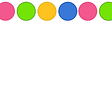# Hyperbolic Floors

Hyperbolic Floors is an interactive visualization that maps hundreds of floors across the world to tessellations in Hyperbolic space. The result is a novel way to look at floor tilings and their infinite nature. The technique is inspired by Hyperbolization of Euclidean Ornaments by Martin von Gagern and combines images sourced from @parisianfloors.

This post describes the project in more detail, going through several examples and implementation details.

# General Approach

The general approach for this project consists of three steps:

1. Classify the symmetry group of the floor pattern.
2. Find a proper mapping from a fundamental domain and a symmetry group in Euclidean space to Hyperbolic space.
3. Render the visualization on the screen.

More specifically, given a picture of a floor, we:

1. Extract its Wallpaper Group and fundamental domain. A Wallpaper Group is a classification of symmetry groups in the 2D plane. The groups classify the tiling of the plane through operations like reflections, gyrations, glide reflections and translations of an initial fundamental domain.
2. Find a suitable mapping from the fundamental domain and group in Euclidean space to Hyperbolic space. We want to find a way to stretch the fundamental domain of a wallpaper group to a fundamental domain of a group in Hyperbolic space such that the new tiling preserves the identity of the floor pattern. We also want to find a suitable symmetry group in hyperbolic space (ie a reflective hyperbolic symmetry group for a reflective euclidean group, a rotational group for a rotational group, and so on).
3. Render the tiling in hyperbolic space. There are infinite copies of the fundamental domain in a hyperbolic tiling, so we need a rendering approach that is not computationally expensive or inaccurate. Here we use the reverse pixel lookup approach introduced by von Gagern and do most of the computations in the fragment shader.

Let’s look at a few examples to better understand the process.

## Reflective groups

For the first example we pick the most common symmetry found in the dataset of @parisianfloors: the *442 symmetry. No need to read much into the orbifold notation if you’re not familiar with it. What’s essential here is that this is primarily a group made of reflected copies of a fundamental domain.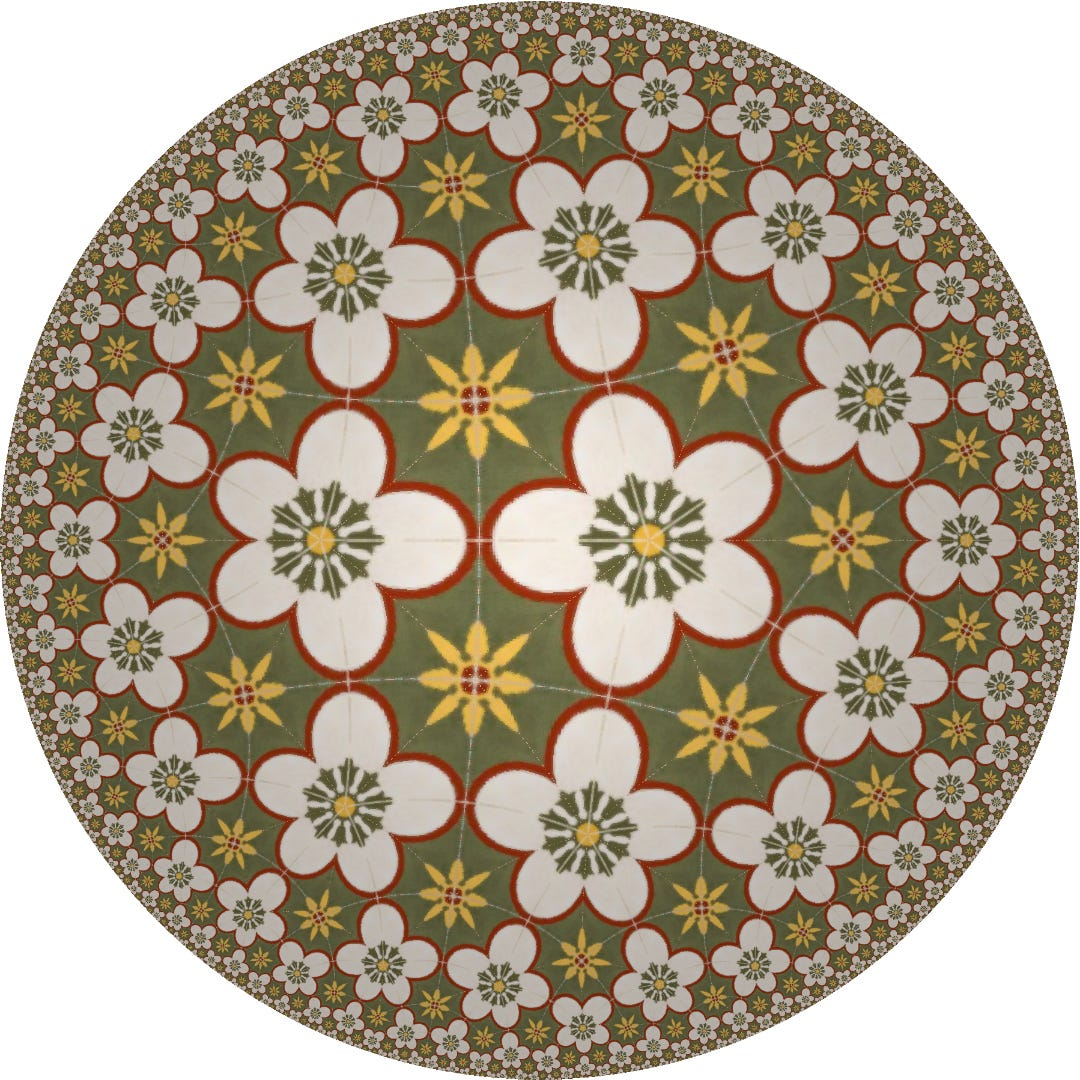Hyperbolization of a *442 floor. This floor has been captured by @parisianfloors at La Serena Altea

The diagram below shows how the fundamental domain (in yellow), gets reflected to generate the tiling. We can also see how this corresponds to the floor’s symmetry below.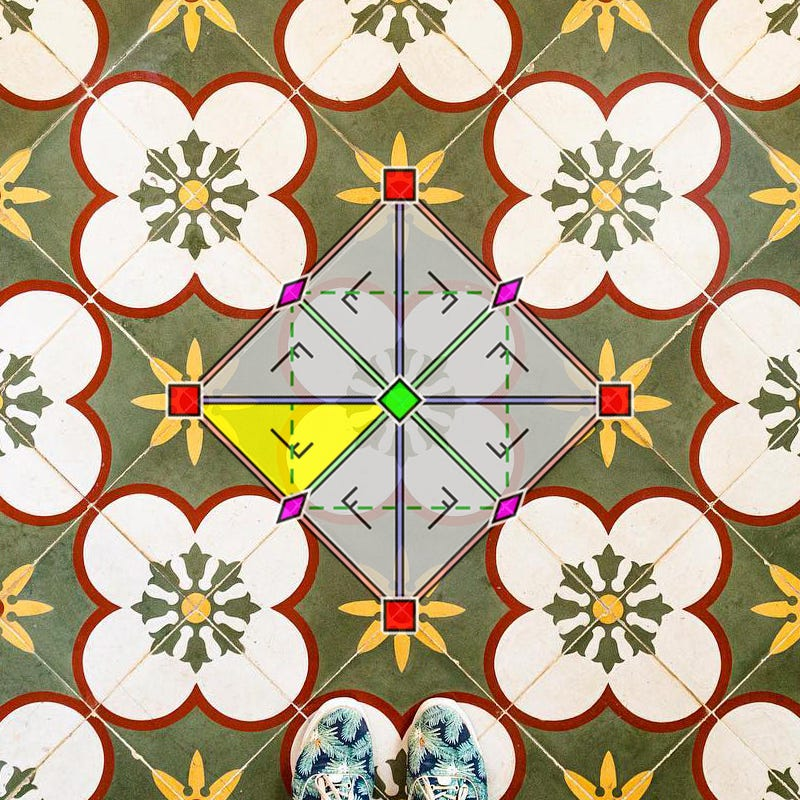Left: the fundamental domain (yellow) gets reflected across four axes. Right: we overlap the relations on the floor.

The goal is then to find a Hyperbolic reflection group where the fundamental domain is a hyperbolic triangle. Here we chose the group {5,4,2}, made of hyperbolic triangles with π/5, π/4 and π/2 angles respectively.

Here’s another example of a reflective group, though less commonly found in floor tilings. The following group is *632:Picture taken by @parisianfloors at Rue du Caire and its Hyperbolization on the right

Again the diagram below shows how the fundamental domain (in yellow), gets reflected over the plane to generate the tiling. We can also see how this corresponds to the floor’s symmetry below. Although the pattern is more convoluted than our previous example, it’s still made of reflections across multiple axes and the fundamental domain is still a triangle.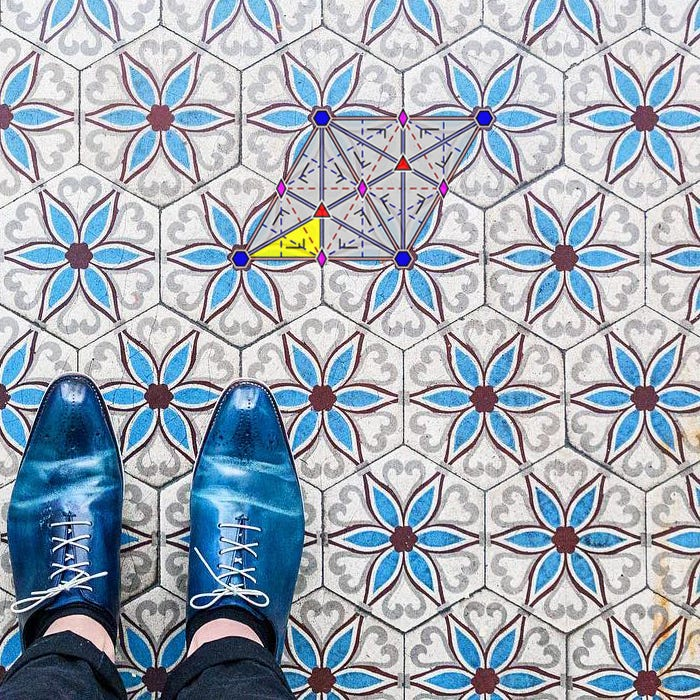Left: the fundamental domain (yellow) and its reflection axes. Right: we overlap the relations on the floor.

This means that the process to generate the hyperbolic tessellation will be the same as in the previous example. We chose {2,7,3} this time which is a triangle of π/2, π/7 and π/3 angles respectively.

Now that we’ve covered the process for reflective groups, let’s look at groups with rotations.

## Groups with rotations

Let’s have a look at 333: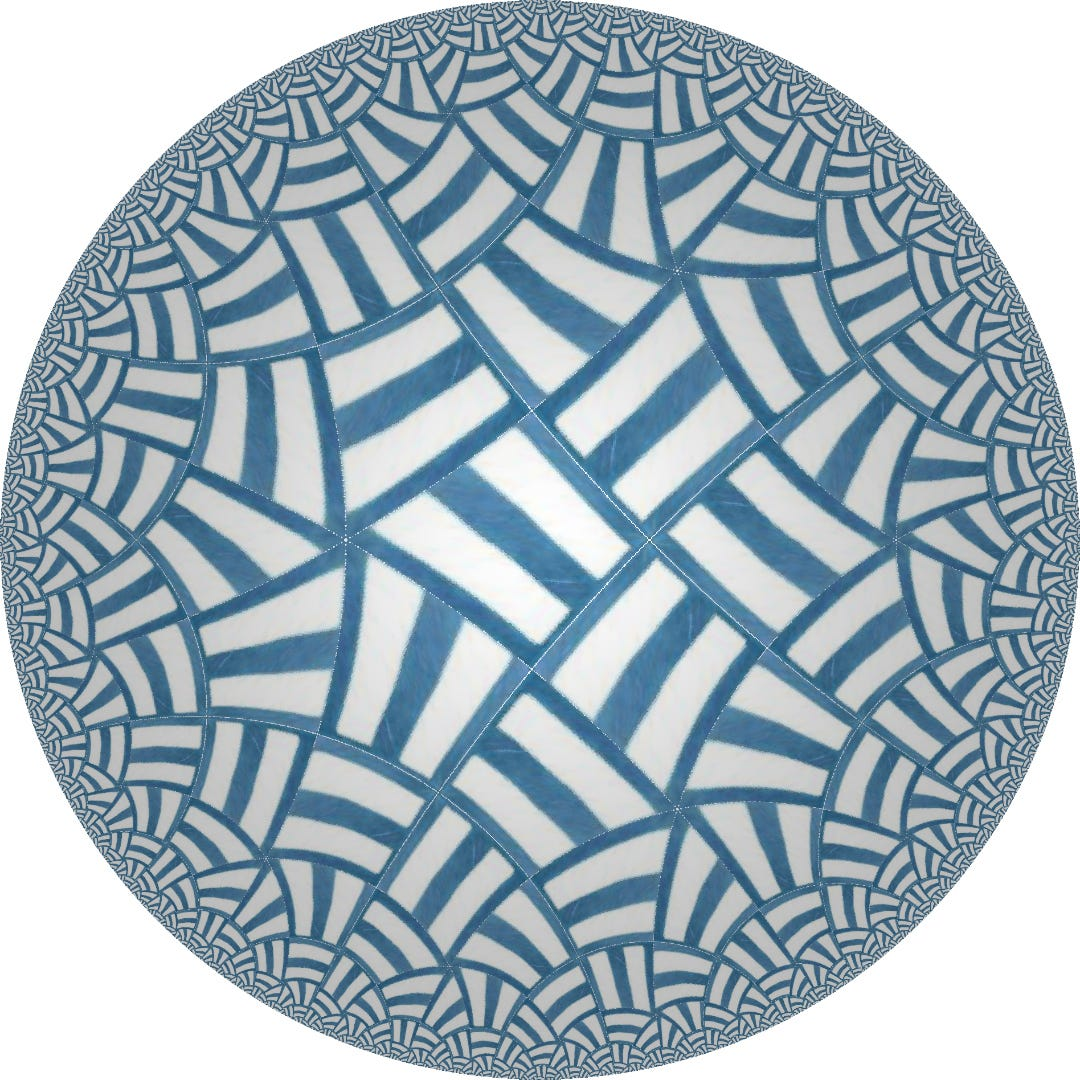Left: Picture taken by @parisianfloors at NAÀN in Paris and its Hyperbolization on the right.

To create this tessellation we rotate the fundamental domain across rotation centers of order three (120°). There are no reflections. The diagram below shows how the fundamental domain (in yellow), gets rotated to generate the tiling. We can also see how this corresponds to the floor’s symmetry below. There’s two main differences with the previous examples:

1. The tiling is not made with reflections but made of rotated copies of the fundamental domain.
2. The fundamental domain is not a triangle but a 4-sided polygon.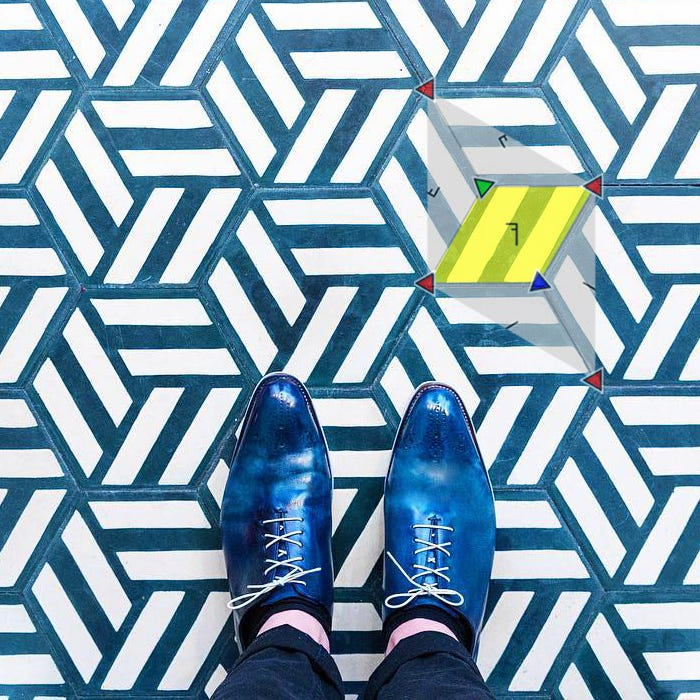Left: the fundamental domain (yellow) and its rotation centers. Right: we overlap the relations on the floor.

For this we need to find a good mapping from the quadrilateral in Euclidean space to a quadrilateral in Hyperbolic space. In addition to this the group in Hyperbolic space must also rotate the fundamental domain instead of reflecting it.

We can create a quadrilateral by combining two adjacent triangles -creating a kite shape. If we do this, then we can still pick a triangle group but having joined pairs of triangles to form the kite shape. In this example we chose the group {4,2,6}, with triangles with angles of π/4, π/2 and π/6. We then join two adjacent triangles to create the polygon we want to achieve to map our fundamental domain.From left to right: combining adjacent triangles in the triangle group {4,2,6} into kites which create the fundamental domain.

Here’s another example of a rotational group, which is the second most common found tiling in @parisianfloors. The group 442. We create the same procedure as before to generate a kite as a fundamental domain of the group. Below you can see the fundamental domain on the picture of the floor as well as in the hyperbolic tiling.

Finally let’s have a look at a group I’ve only seen once in the @parisianfloors dataset: 632.

This group is tricky because it’s a rotation group (the fundamental domain gets rotated multiple times to cover the plane) but the fundamental domain for this group is a triangle instead of a quadrilateral as in the previous examples.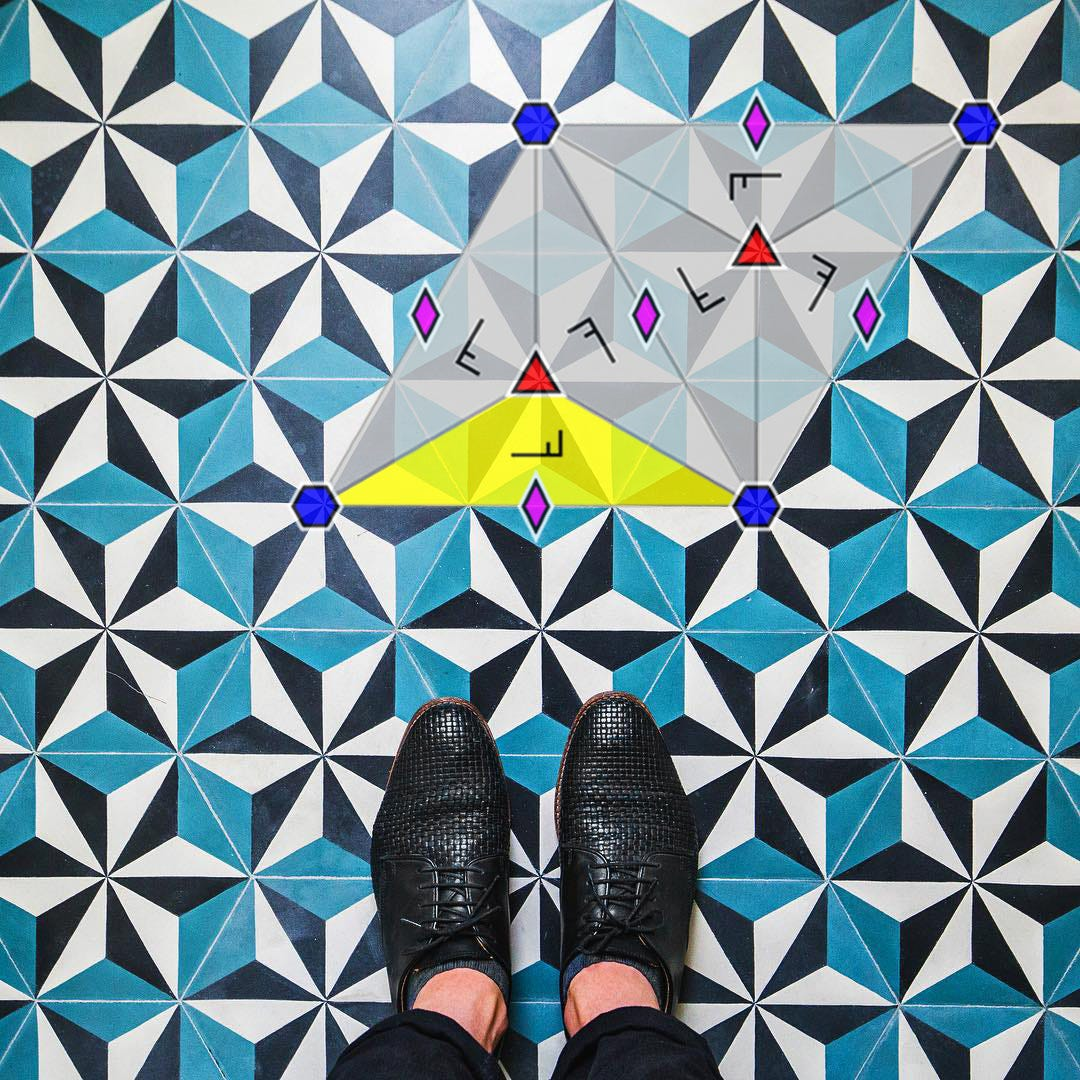Left: the fundamental domain (yellow) and its rotation centers. Right: we overlap the relations on the floor.

To work around this we take as fundamental domain for the Hyperbolic group the two stitched triangles at the center of the motif in the above picture, making it a quadrilateral and treating this as if it was the 333 example above.

Congratulations on getting to this point! It means you grasped the work needed to map from a wallpaper group to a hyperbolic group. It may be apparent also that each wallpaper group has its own intricacies and needs to be evaluated individually. There are 17 groups and for some of them the Hyperbolization process gets really challenging.

By now you may also have three unanswered questions:

1. How do we scale this process? Especially how do we scale wallpaper group classification and fundamental domain extraction?
2. How is the mapping done between fundamental domains? Is it a conformal mapping? This step is key to getting the right output.
3. How do you render this on the screen? How does reverse pixel lookup actually work?

I will try to answer the following questions next through implementation notes. This might get more granular and uninteresting, so feel free to stop reading now :)

# Implementation Notes

## Group classification and fundamental domain extraction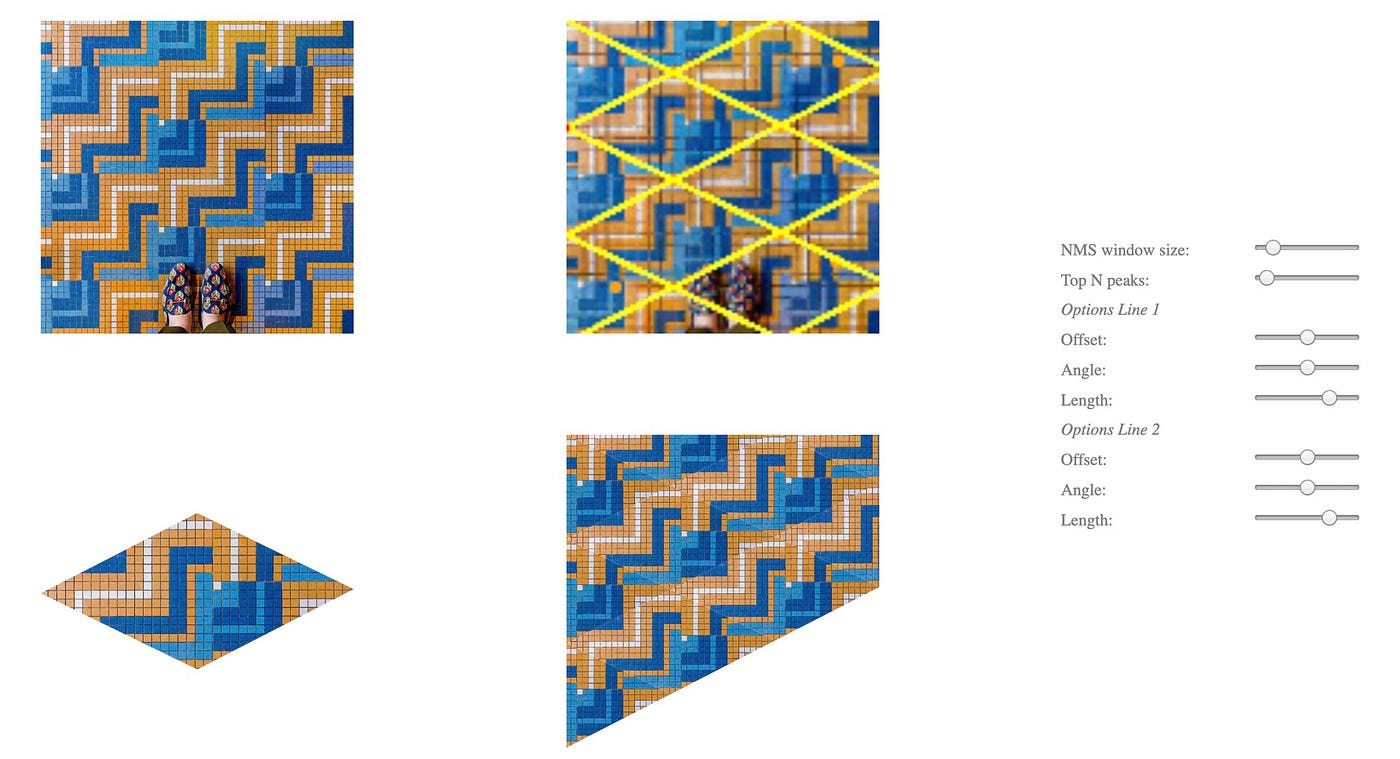A user interface that extracts the unit tile of a picture. Top-left: original image. Top-right: axes extracted from Hough Transform, Bottom-left: Unit tile, Bottom-right: reconstructed floor from extracted unit tile.

In his paper Martin von Gagern mentions that a fully automated way of detecting groups and extracting fundamental domains from Euclidean ornaments was built. The work is based on two papers:

In both papers the process is as follows. Given an input image:

1. We compute the autocorrelation of the image.
2. From the autocorrelated image we select peaks based on non-maximum suppression.
3. We feed the output to the generalized Hough transform to obtain two vectors (shown as the yellow lines in the image above).

If all this goes well, we are able to extract the unit tile from the floor pattern. The unit tile is not the fundamental domain: the unit tile is the area which can generate the floor pattern by only using translations. It usually is made of multiple fundamental domains stitched together.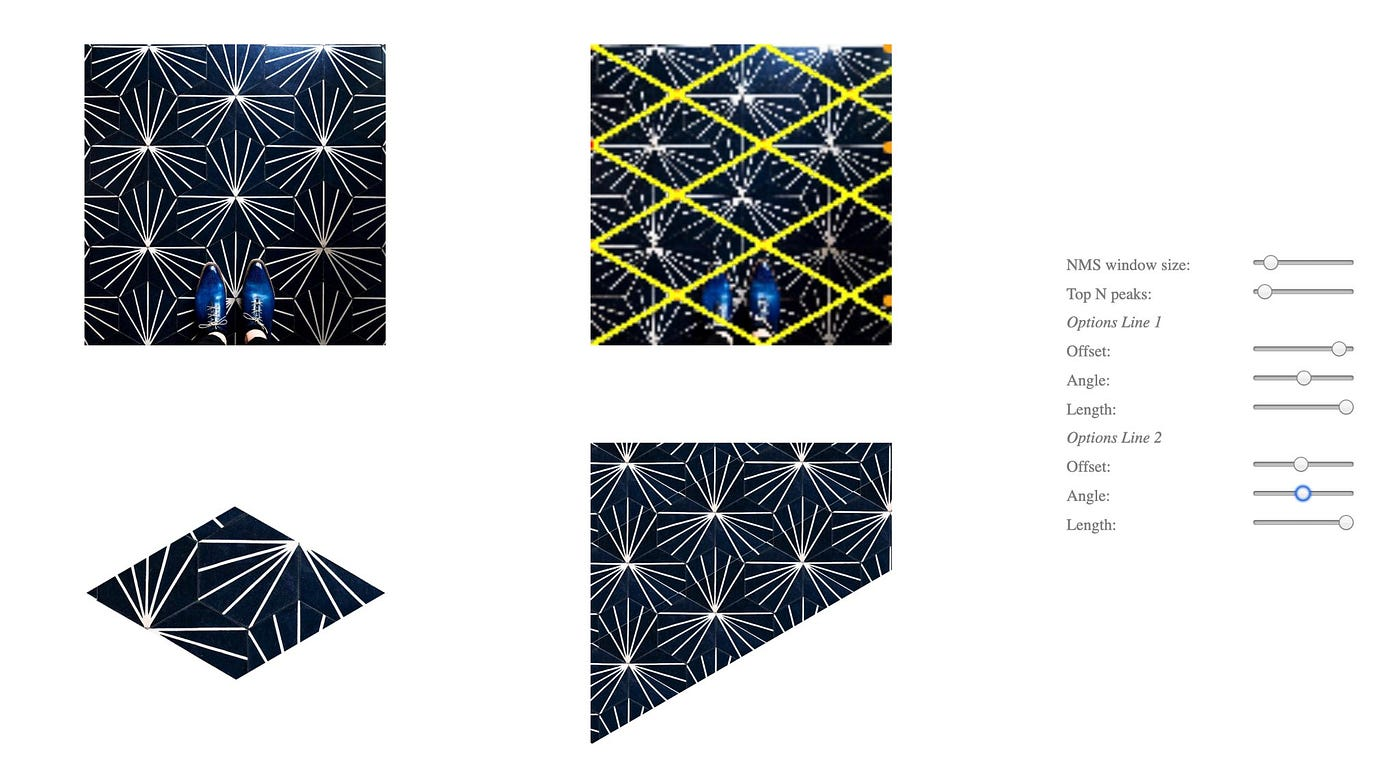The bottom left image is the unit tile of the floor pattern extracted through the process described above. With the vectors returned by the Hough transform (top-right) and the unit tile (bottom-left) we can reconstruct the floor pattern (bottom-right).

Once we obtain the unit tile, next step is to register this tile with other tiles found in the original image using sum square difference (SSD) to understand how much the tiles vary from each other in the image.

With this as reference we can test out correlating the original image with reflected and rotated (at different angles) versions of the unit tile. This will reveal what symmetries the image exhibits, and deterministically extract its fundamental domain.

After working on a full implementation however, this process worked for me about 60% of the time. The main challenge lies with finding the right peaks in the autocorrelated image. Although I have not spoken with the author, von Gagern writes in his paper that “The heuristics used to extract basis vectors from this fuzzy grid of peaks are still under development”.

It seems like there is an opportunity for improvement on this specific step of the hyperbolization process. One possibility to explore is to build an ML classifier instead of going the full deterministic / brute force route.

I opted for an assistive method, building a user-interface that would let me quickly classify the floor tiling and extract its fundamental domain. This introduced quite a bit of human error but I still believe was the right tradeoff between time and quality. We ran this through 750+ posts generating 240+ hyperbolizations. The interface averages unit tiles to get better quality tiles to be used in the hyperbolization.

## Mapping fundamental domains

A key step to be able to find the right hyperbolic representation of a Euclidean ornament is to map the fundamental domain from Euclidean to Hyperbolic space.

von Gagern uses the method in Conformal Equivalence of Triangle Meshes by Springborn et al. to achieve a conformal mapping between domains. This paper relies on the definition of discrete conformal equivalence between two metrics to solve a convex optimization problem. The author makes use of the C++ library PETSc/TAO to solve this optimization problem. While this paper provides a suitable solution, we are looking for something that can be fully implemented in JavaScript, can be done in real-time and only works for domains in 2D space. So I started looking for a paper that might provide a simpler approach to achieve this mapping.

That’s when I came to Iterative Closest Conformal Maps between Planar Domains by Aviv Segall et al. The authors also provide code in Matlab for this algorithm. The paper makes use of the Cauchy Transform and the Cauchy-Green coordinates to find a conformal mapping between two domains. Given a mapping ƒ(w) between boundaries of two domains, the Cauchy Transform provides the space of holomorphic functions across the domains themselves:Note that although ƒ is defined in ∂Ω, u(z) is defined for all of Ω.

The paper then makes use of the Cauchy coordinates which are a discretized version of the integral. We sample with n points the boundaries of source and target domains, and the algorithm will provide a matrix that can be leveraged with any point in(side) the source domain to find the right mapping to its target.

While the paper described above is a suitable implementation, can be done in JavaScript and the coefficients can be uploaded as a uniform array into the GPU for real-time computation of the mappings, I found another paper with a much simpler (but not conformal) approach to mapping between boundaries: Bending Circle Limits by Vladimir Bulatov. The mapping is simply a linear combination between a source domain and a unit square and back to a target domain.

This approach is easy to implement in both JavaScript and GLSL and the results are very promising as can be seen in the paper. We use this paper as the basis for our mapping algorithm.

For each Wallpaper / Hyperbolic group pair, we render to a texture the Hyperbolic fundamental domain using as colors the uv mapping to the Euclidean fundamental domain. We then upload two textures when rendering the final hyperbolic ornament: the unit tile itself, and the uv mapping to the euclidean domain. For each pixel in screen space we perform two lookups. First the mapping over to the coordinates in the unit tile, and then the color for the pixel in the unit tile itself.

## Reverse pixel lookup

As mentioned in the beginning of this post, we implement the reverse pixel lookup technique by von Gagern to render the hyperbolic tessellation in the screen. Instead of starting with an initial fundamental domain and applying transformations to tessellate it, this technique reverses the flow starting from points in screen space and repeatedly applying group elements until the location is within the central domain. This makes the algorithm also very suited for a fragment shader implementation.

In the case of a reflective hyperbolic group which has a triangle as a fundamental domain, given a point in screen space we “fold” (reflect) the point across the edges of the triangle continuously until we get to the central fundamental domain.

For a full implementation in GLSL you can read Generating spherical and hyperbolic tilings in GLSL from Roy’s blog, in which he also covers the approach to Euclidean and Spherical geometries.

# Conclusion

That’s a wrap! We presented an interactive visualization which maps floor tilings to hyperbolic symmetry groups. We talked about the general approach with several examples and then did a deep dive with a few implementation notes on each step of the process.

There are several things that remain unsolved. In particular there are 17 Wallpaper groups and we haven’t implemented mappings for all of them to Hyperbolic space. Each group is different and this is where most of the craft lies for this work.

Another extension proposed by von Gagern was to implement a similar approach for Spherical groups. This could be an interesting path to explore next.

--

--

--

## More from Nicolas Belmonte

Interested in Computational Design and Generative / Math Art.

Love podcasts or audiobooks? Learn on the go with our new app.

## The formula of the Universe is for suckers!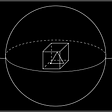## Powers of Two: Ones, Tens, and Hundreds of Digits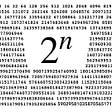## Why is the Bayesian Theorem different from the frequentist statistic approach?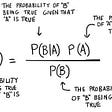## Law of Conservation of Momentum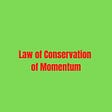## General Topology — Part 2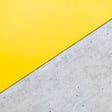## QC — Observable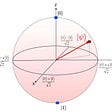## Fantastical Primes and Where to Find Them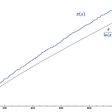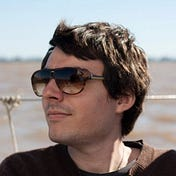## Nicolas Belmonte

Interested in Computational Design and Generative / Math Art.

## The size of the meteorite that caused the extinction of the dinosaurs is… quite unexpected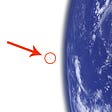## A Proposal for a Conceptor Calculus, Formal Unity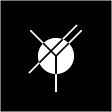## Beer catalyzed civilization. Beer is the key to space colonization.## Division Definition, Symbol, Examples